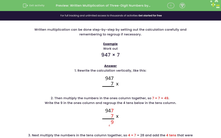# Multiply Three-Digit Numbers by One-Digit Numbers Using Carrying

In this worksheet, students will carry out formal written multiplications of three-digit numbers by single-digit numbers. Carrying is involved.Key stage:  KS 2

Curriculum topic:   Number: Multiplication and Division

Curriculum subtopic:   Use Written Form for Multiplication

Difficulty level:#### Worksheet Overview

Written multiplication can be done step-by-step by setting out the calculation carefully and remembering to carry numbers if necessary.

Example

Work out:

947 × 7

Rewrite the calculation vertically, like this:Multiply the numbers in the ones column together, so 7 × 7 = 49

Write the 9 in the ones column and carry the 4 tens below to the doorstep of the tens column.Next, multiply the numbers in the tens column together, so 4 × 7 = 28 and add the 4 tens that were on the doorstep.This gives us 28 + 4 = 32 , so we write the 2 in the tens column and carry the 3 hundreds below on to the doorstep of the hundreds column.Lastly, multiply the numbers in the hundreds column, so 9 × 7 = 63 and add the 3 that was on the doorstep.

This gives us 63 + 3 = 66 in the thousands.So, in full, we have:So      947 × 7 = 6,629

Are you ready to have a go at some questions yourself? You can look back at this example at any point by clicking on the red help button that appears on the screen during the questions.### What is EdPlace?

We're your National Curriculum aligned online education content provider helping each child succeed in English, maths and science from year 1 to GCSE. With an EdPlace account you’ll be able to track and measure progress, helping each child achieve their best. We build confidence and attainment by personalising each child’s learning at a level that suits them.

Get started